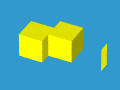# Regularized Boolean OperatorsWe certainly expect that the union, intersection and difference of two solids is a solid. Unfortunately, in many cases this is not always true. In the following figure, two cubes touch each other and their intersection is a rectangle shown on the right. A rectangle is not a three-dimensional object and hence not a solid!To eliminate these lower dimensional branches, the three set operations are regularized as follows. The idea of regularing is very simple.

• Compute the result as usual and lower dimensional components many be generated.
• Compute the interior of the result. This step removes all lower dimensional components. An example has been shown in previous page. The result is a solid without its boundary.
• Compute the closure of the result obtained in the above step. This adds the boundary back.

Let +, ^ and - be the regularized set union, intersection and difference operators. Let A and B be two solids. Then, A + B, A ^ B and A - B can be defined mathematically based on the above description:

A + B = closure(int(the set union of A and B)
A ^ B = closure(int(the set intersection of A and B)
A - B = closure(int(the set difference of A and B)
where closure() and int() are the closure and interior discussed in previous page. Based on this definition, the intersection of the two cubes shown at the beginning of this page is empty. As mentioned earlier, the set intersection of these two cubes is a rectangle, which is a two dimensional object and has no interior. Hence, after taking interior (i.e., int()), we get an empty set, whose closure is also empty. Consequently, the intersection is empty.